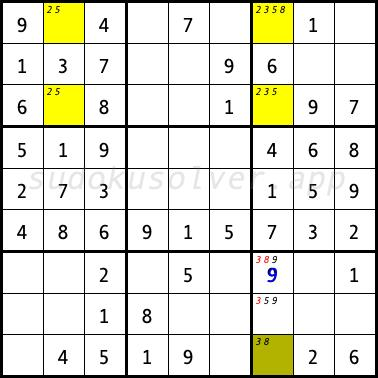# Type 3 Unique Rectangle

The Type 3 Unique Rectangle combines a unique rectangle and a naked subset for additional candidates. In the sudoku diagram below, a non-unique rectangle can appear in the cells R1C2, R1C7, R3C2 and R3C7 with the digits 2 and 5. The cells R1C7 and R3C7 have additional candidates, the digits 3 and 8. To prevent a non-unique rectangle, one of these cells must take one of these additional digits. The cell R9C7 has exactly two candidates 3 and 8. If this cell had the digit 3 then the cell R1C7 would have to have the digit 8. If the cell R9C7 had the digit 8 then one of the cells R1C7 or R3C7 would have to have the digit 3. Therefore these two digits 3 and 8 cannot be placed in other cells than R1C7, R3C7 and R9C7. So we can eliminate these digits from the remaining cells of the seventh column. Thanks to this elimination, we can place the digit 9 in the R7C7 cell.